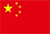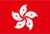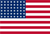Scanning QR Code• Online Inquiry

+
Congratulations
You've successfully got advanced quotes worth 5000 HKD
• Home
• Quotes
• News
• Learn
• Help Center
• Language
•••EN

# RSI relative strength indicator introduction

## 1. Introduction

The relative strength index is a momentum indicator used in technical analysis that measures the magnitude of recent price changes to evaluate overbought or oversold conditions in the price of a stock or other asset. The RSI is displayed as an oscillator (a line graph that moves between two extremes) and can have a reading from 0 to 100. The RSI is calculated as the ratio of the average value of the total price increase to the average value of the total price increase and decrease within a certain period of time. RSI1, RSI2, and RSI3 correspond to the 6th, 12th, and 24th respectively.

## 2. Calculation formula

RSI (N day) = 100% * (Sum of closing increase in N days) / (Sum of absolute value of closing increase and decrease in N days)

## 3. Application

3.1 The RSI value is between 0 and 100, and the distribution is normal. The most common variablesare the 6, 12, and 30-day RSI, which are used to gaugeshort-term and mid-term market trends, respectively.

3.2 RSI is a stock price analysis technical tool based on relative rise and fallof the price action. Generally, RSI is at 50 as the trading equilibrium point, and 30 to 70 is the normal trading state.

3.3 On the 6-day basis chart, if the RSI is above 80 or below 15, a reversal signal may appear.

3.4 If RSI is greater than 80, the price would enter the overbought zone and should be sold in batches; if RSI is greater than 90, it's sign of a severely overbought, the stock price will go back at any time, and it is recommended to sell the shares.

3.5 RSI is less than 20,the price has enteredthe oversold zone, you should buy in batches; RSI is less than 10, which means that it is seriously oversold and traders should go for a dip.

## 4. Features

4.1 RSI is normally allocated and can be used to determine the overbought or oversold phenomenon of the stock market.

4.2 RSI will peak or bottom before stock price changes, which can reflect the ups and downs of stock prices in advance and can be regarded as a leading indicator of the trend of the market index.

4.3 RSI is a stock price momentum strength indicator. The high and low points formed by its trajectory can be used as tangent and tangent points to find pressure and support lines.

4.4 The RSI value can be regarded as an indicator of the strength of long and short positions to grasp the progress of the trend. Taking the RSI value of 50 as the equilibrium point of the long-short battle, when the RSI value is above 50 for a long time, it is a long uptrend; when the RSI value is below 50 for a long time, it is a short downtrend. The rising or falling direction of the RSI line represents a change in the momentum of long and short positions.

4.5 The trend of the RSI line deviates from the trend of the market index, which means that the market is about to reverse.

4.6 RSI could fail if it is sitting at extremely high or low quotes.

Back to the Top